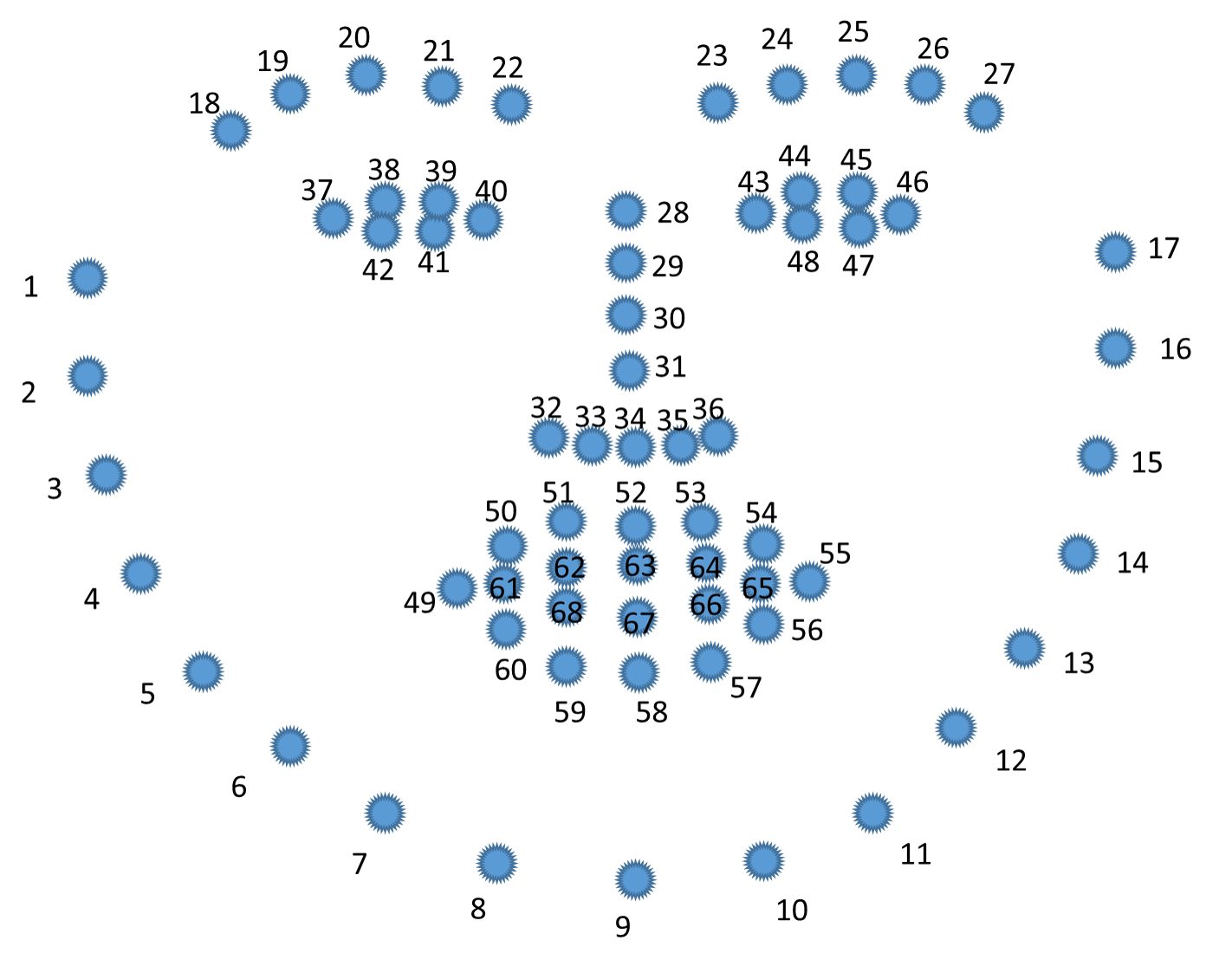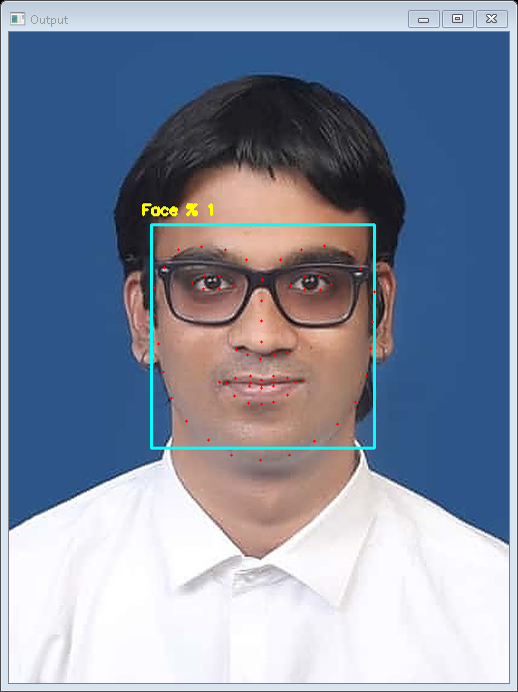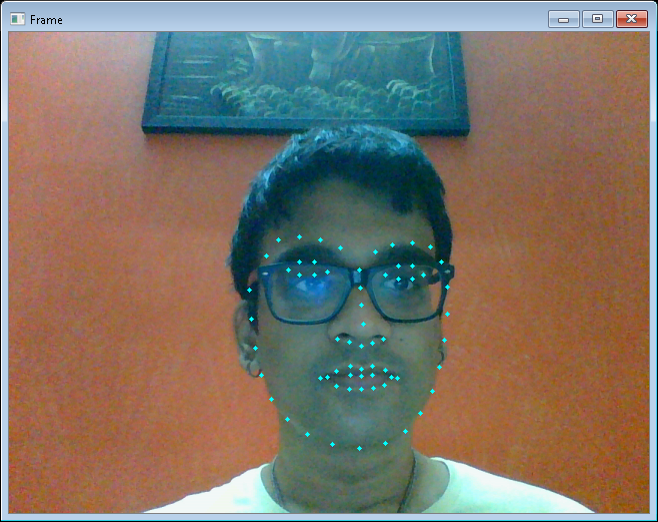# OpenCV – Facial Landmarks and Face Detection using dlib and OpenCV

The world will be taken over by Artificial Intelligence very soon. Face Applications include identification of faces from videos or digital images. It is a good example of Computer Vision. For Identification with better accuracy and confidential value, the faces need to be detected properly. Face Detection Technology is used in applications to detect faces from digital images and videos. Also, just detecting the face will not help. We need more information about the face, i.e. whether a person smiles, laughs, or dimples seen while smiling etc. In short, facial expressions too give us information. In order to get more information about the face, we take the help of Facial Landmarks.

What are Facial Landmarks?
Facial landmarks are used for localizing and representing salient regions or facial parts of the person’s face, such as:

• Eyebrows
• Eyes
• Jaws
• Nose
• Mouth etc.

Facial landmarks is a technique which can be applied to applications like face alignment, head pose estimation, face swapping, blink detection, drowsiness detection, etc.

In this context of facial landmarks, our vital aim is to detect facial structures on the person’s face using a method called shape prediction.
Facial Landmarks Detection has 2 steps:

1. To detect the key facial structures on the person’s face.
2. It involves localizing the face in the image.

We can do Face detection in a number of ways. We can use OpenCV’s built-in Haar Cascade XML files or even TensorFlow or using Keras. Over here especially, We need to apply a HOG (Histogram of Gradients) and Linear SVM (Support Vector Machines) object detector specifically for the task of face detection. We can also do it using Deep Learning-based algorithms which are built for face localization. Also, The algorithm will be used for the detection of the faces in the image. We can obtain face bounding box through some method for which we use the (x, y) coordinates of the face in the image respectively.
We do have a variety of facial landmark detectors, but every method will essentially be trying to localize and also labelling the following facial regions will be done.

• Nose
• Jaws
• Left eye
• Right eye
• Left eyebrow
• Mouth
• Right eyebrow

The facial landmark detector which is pre-trained inside the dlib library of python for detecting landmarks, is used to estimate the location of 68 points or (x, y) coordinates which map to the facial structures. These indexes of 68 coordinates or points can be easily visualized on the image below:The Locations of the Facial Parts are as follows:

• The left eye is accessed with points [42, 47].
• The mouth is accessed through points [48, 67].
• The left eyebrow is accessed through points [22, 26].
• The nose is accessed using points [27, 34].
• The right eyebrow is accessed through points [17, 21].
• The right eye is accessed using points [36, 41].
• And the jaw is accessed via points [0, 16].

Following are the steps for Implementation of Face Landmarks Detection:

• Install Python 3. Also Spyder terminal, Jupyter Notebook or Pycharm Editor recommended.
• Install libraries imutils, argparse, numpy, dlib and cv2-contrib-python and cv2-python using pip(Windows) and sudo apt for Linux.
• Download the dlib shape predictor. It is a file with .dat extension.

Code: Implementation of Facial Detection with Facial Landmarks using Python

 `# We import the necessary packages ` `from` `imutils ``import` `face_utils ` `import` `numpy as np ` `import` `argparse ` `import` `imutils ` `import` `dlib ` `import` `cv2 ` ` `  `# We construct the argument parser and parse the arguments ` `ap ``=` `argparse.ArgumentParser() ` `ap.add_argument(``"-p"``, ``"--shape-predictor"``, required ``=` `True``, ` `    ``help` `=``"path to facial landmark predictor"``) ` `ap.add_argument(``"-i"``, ``"--image"``, required ``=` `True``, ` `    ``help` `=``"path to input image"``) ` `args ``=` `vars``(ap.parse_args()) ` ` `  `# We are initializing the  dlib's face detector (HOG-based) and then  ` `# creation of the facial landmark predictor ` `detector ``=` `dlib.get_frontal_face_detector() ` `predictor ``=` `dlib.shape_predictor(args[``"shape_predictor"``]) ` ` `  `# We then load the input image, resize it, and convert it to grayscale ` `images ``=` `cv2.imread(args[``"image"``]) ` `images ``=` `imutils.resize(images, width ``=` `500``) ` `gray ``=` `cv2.cvtColor(images, cv2.COLOR_BGR2GRAY) ` ` `  `# We then detect faces in the grayscale image ` `rects ``=` `detector(gray, ``1``) ` ` `  `# Now, job is to loop over the face detections ` `for` `(i, rect) ``in` `enumerate``(rects): ` `    ``# We will determine the facial landmarks for the face region, then ` `    ``# can convert the facial landmark (x, y)-coordinates to a NumPy array ` `    ``shape ``=` `predictor(gray, rect) ` `    ``shape ``=` `face_utils.shape_to_np(shape) ` ` `  `    ``# We then convert dlib's rectangle to a OpenCV-style bounding box ` `    ``# [i.e., (x, y, w, h)], then can draw the face bounding box ` `    ``(x, y, w, h) ``=` `face_utils.rect_to_bb(rect) ` `    ``cv2.rectangle(images, (x, y), (x ``+` `w, y ``+` `h), (``255``, ``255``, ``0``), ``2``) ` ` `  `    ``# We then show the face number  ` `    ``cv2.putText(images, ``'Face % {}'``.``format``(i ``+` `1``), (x ``-` `10``, y ``-` `10``), ` `        ``cv2.FONT_HERSHEY_SIMPLEX, ``0.5``, (``0``, ``255``, ``255``), ``2``) ` ` `  `    ``# We then loop over the (x, y)-coordinates for the facial landmarks  ` `    ``# and draw them on the image ` `    ``for` `(x, y) ``in` `shape: ` `        ``cv2.circle(images, (x, y), ``1``, (``0``, ``0``, ``255``), ``-``1``) ` ` `  `# Now show the output image with the face detections as well as  ` `# facial landmarks ` `cv2.imshow(``"Output"``, images) ` `cv2.waitKey(``0``) `

Steps for the Output Generation:

• Open the Command prompt
• Go to the path where this program is saved. Also save the image for landmark detection of faces in the same path or you can save the image in another folder but that folder should be saved in the same path

• Syntax for the command:-
`python filename.py ––shape-predictor shape_predictor_68_face_landmarks.dat ––image image_folder_name/image_name.file extension` (This is if the image is saved in a separate folder )
• Syntax for the command:-
`python filename.py ––shape-predictor shape_predictor_68_face_landmarks.dat ––image image.file extension` (This is if the image is saved in a same path where program is saved)
• Insert the command for the execution.
Here, the image is saved in the same path but separate folder.
```python facial_landmarks.py ––shape-predictor shape_predictor_68_face_landmarks.dat –– image images/Vignesh.jpg```.As seen in the Output, the Landmarks are shown in red color dots and the Face Detection is in Cyan color box drawn around the face.
(Note:- The above steps for execution works for Windows and Linux.)

Code: Implementation of Facial Landmarks with Real Time using Python

 `# We Import the necessary packages needed ` `import` `cv2 ` `import` `numpy as np ` `import` `dlib ` ` `  `cap ``=` `cv2.VideoCapture(``0``) ` `# We initialise detector of dlib ` `detector ``=` `dlib.get_frontal_face_detector() ` `predictor ``=` `dlib.shape_predictor(``"shape_predictor_68_face_landmarks.dat"``) ` ` `  `# Start the main program ` `while` `True``: ` `    ``_, frame ``=` `cap.read() ` `# We actually Convert to grayscale conversion ` `    ``gray ``=` `cv2.cvtColor(frame, cv2.COLOR_BGR2GRAY) ` `    ``faces ``=` `detector(gray) ` `    ``for` `face ``in` `faces: ` `   ``# The face landmarks code begins from here ` `        ``x1 ``=` `face.left() ` `        ``y1 ``=` `face.top() ` `        ``x2 ``=` `face.right() ` `        ``y2 ``=` `face.bottom() ` `        ``# Then we can also do cv2.rectangle function (frame, (x1, y1), (x2, y2), (0, 255, 0), 3) ` `        ``landmarks ``=` `predictor(gray, face) ` `       ``# We are then accesing the landmark points  ` `        ``for` `n ``in` `range``(``0``, ``68``): ` `            ``x ``=` `landmarks.part(n).x ` `            ``y ``=` `landmarks.part(n).y ` `            ``cv2.circle(frame, (x, y), ``2``, (``255``, ``255``, ``0``), ``-``1``) ` `    ``cv2.imshow(``"Frame"``, frame) ` `    ``key ``=` `cv2.waitKey(``1``) ` `    ``if` `key ``=``=` `27``: ` `        ``break` `# press esc the frame is destroyed `

Steps for Output Generation:

• Open Command Prompt.
Go to the path where this program is saved.
• Syntax of the command is as follows:-
`python filename.py `
• Insert the command for the execution.
`python face_landmarks.py `
•As seen in the Output, the Landmarks are shown in Cyan color dots.
(Note:- The above steps for execution works for Windows and Linux.)
References:

My Personal Notes arrow_drop_upCheck out this Author's contributed articles.

If you like GeeksforGeeks and would like to contribute, you can also write an article using contribute.geeksforgeeks.org or mail your article to contribute@geeksforgeeks.org. See your article appearing on the GeeksforGeeks main page and help other Geeks.

Please Improve this article if you find anything incorrect by clicking on the "Improve Article" button below.

Article Tags :

1

Please write to us at contribute@geeksforgeeks.org to report any issue with the above content.